Question

Program C:

Start by printing out your name, then your code should prompt the user to enter integers using the following prompt:

Enter some integers, end with a 0:

Level 1 Task: count the number of integers entered (not including the 0)

Level 2 Task: produce the sum of all of the integers

Level 3 Task: produce the sum of the even integers

These calculated values need to be displayed as follows: There were ___ numbers read in (not counting the 0). The sum of the numbers is ___. The sum of the even numbers in the collection of numbers is ____. The blanks should be replaced with the values you calculated based on the input. You can assume only integers will be provided as input.

//note:- if you have any query regarding this then comment below. please upvote

#include<stdio.h>
int main()
{
int arr;
int i=0;
int sum=0,even=0;
printf("Enter some integers, end with a 0: \n");
while(1)
{
int num;
scanf("%d",&num);
if(num==0)
break;
else
{
arr[i]=num;
}
i++;
}
int j;
for(j=0;j<i;j++)
{
if(arr[j]%2==0)
{

even+=arr[j];
}
sum+=arr[j];
}
printf("There were %d numbers read in (not counting the 0)\n.",i);
printf("The sum of the numbers is %d\n.",sum);
printf("The sum of the even numbers in the collection of numbers is %d\n.",even);

}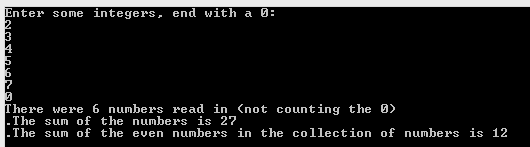#### Earn Coins

Coins can be redeemed for fabulous gifts.

Similar Homework Help Questions
• ### 1. Write a program called Numbers that a. prompts the user for a file name. b....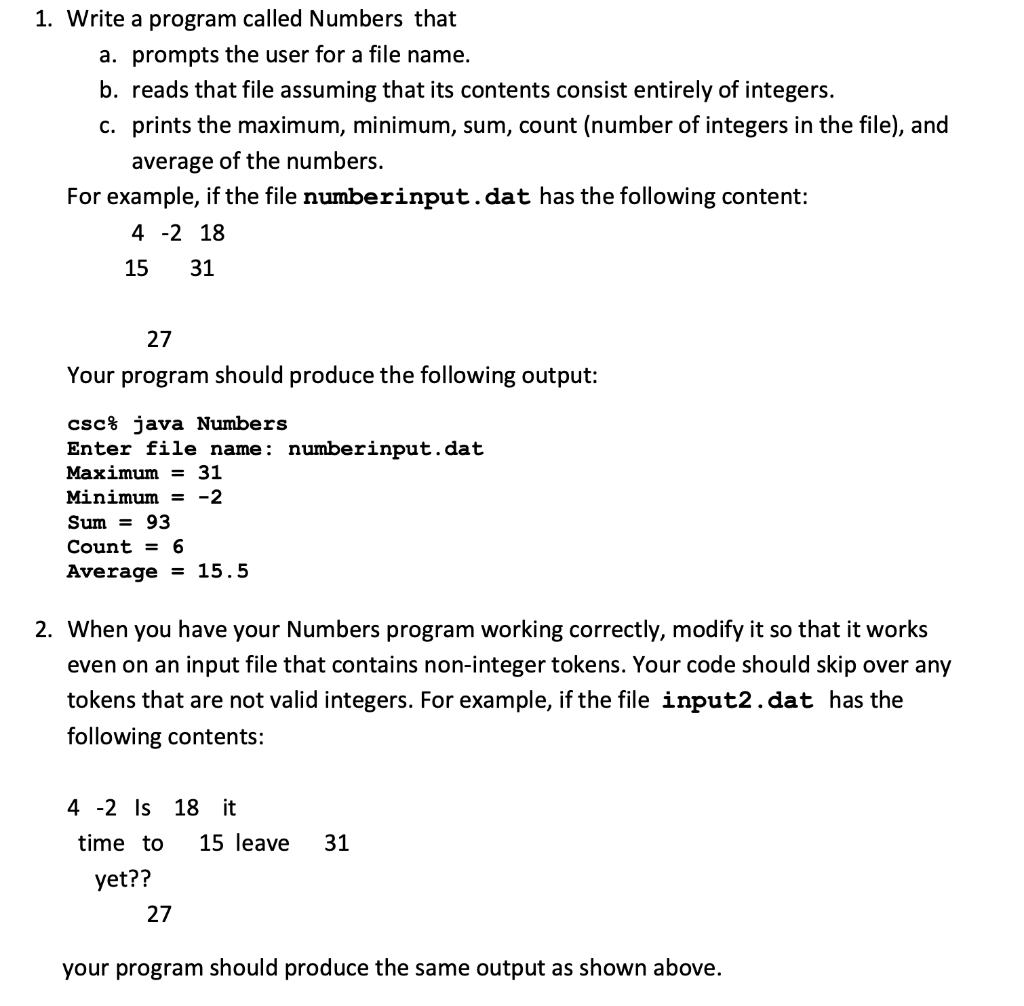1. Write a program called Numbers that a. prompts the user for a file name. b. reads that file assuming that its contents consist entirely of integers. c. prints the maximum, minimum, sum, count (number of integers in the file), and average of the numbers. For example, if the file numberinput.dat has the following content: 4 -2 18 15 31 27 Your program should produce the following output: csc% java Numbers Enter file name: numberinput.daft Maximum31 Minimum- -2 Sum -...

• ### Then it should prompt the user for the input file name of the file containing the data to sort

2. Then it should prompt the user for the input file name of the file containing the data to sort. 3. If the program can't open the file or if no file name is entered (^d or ^z is entered for EOF), it should print an error message and exit. 4. The file will contain numbers that could be positive or negative integers or floating point numbers two numbers to a line. 5. The program should only handle the first...

• ### Write a C program code for the scenerio below: An important device carried up to the...

Write a C program code for the scenerio below: An important device carried up to the International Space Station on the last scheduled flight of space shuttle Endeavor was the Alpha Magnetic Spectrometer (AMS-02). As explained by NASA, the AMS is a particle physics detector, searching for antimatter and dark matter and also measuring cosmic rays. The name strangelets refers to three types of quarks (named u, d or s) which have been discovered experimentally to make up matter, but...

• ### write a programming code for the following problem using Visual Basic Studio VB. Write a program that will allow the user to enter series of mumbers and will give some useful statistics about the mam...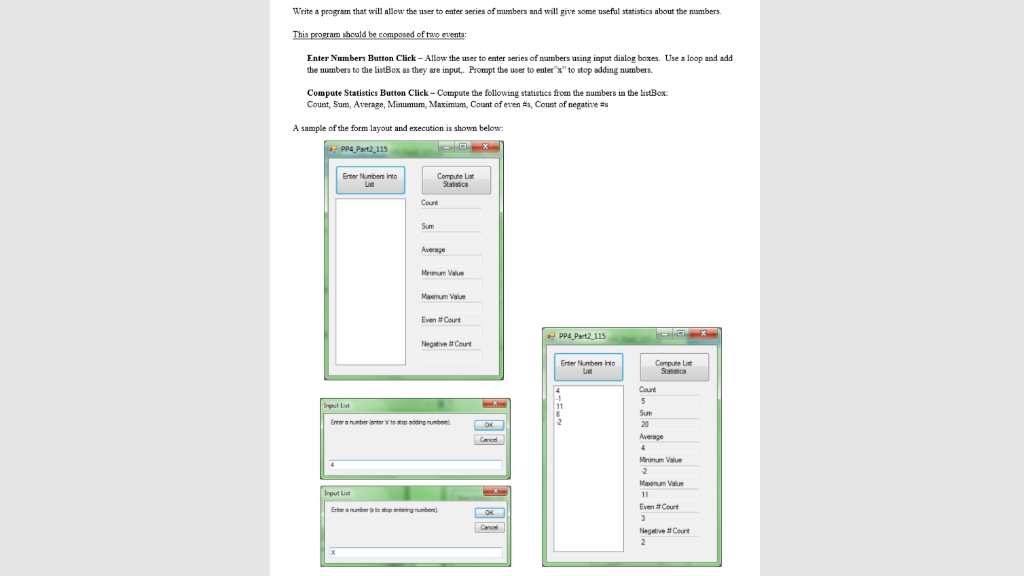write a programming code for the following problem using Visual Basic Studio VB. Write a program that will allow the user to enter series of mumbers and will give some useful statistics about the mambers Enter Numbers Button Click-Allow the user to enter series of nambers using imput dialog boxes. Use a loop and add the numbers to the listBox as they are input.. Prompt the user to enter"x" to stop addang numbers. Compute Statistics Button Click-Compute the following statistics...

• ### Write a program that will loop ten times. In each iteration prompt user to enter an...

Write a program that will loop ten times. In each iteration prompt user to enter an integer between -50&50. Print the input, keep a running sum of the inputs, and track the minimun and maximum numbers input. After the loop is complete, minimum number entered, maximum number entered, sum of all numberes entered, and average of all numbers entered. Format all output. Before running the loop, print the label "Input values: ". Within the loop, print value entered by user...

• ### the user should be prompted to enter an integer and then your program will produce another...

the user should be prompted to enter an integer and then your program will produce another integer depending on whether the input was even or odd. The following is an example of what you might see when you run the program; the input you type is shown in green (press Enter at the end of a line), and the output generated by the program is shown in black text. Enter an integer: 1234 Number is even, taking every other digit...

• ### How do I program this problem using c++. Create a process to prompt the user to...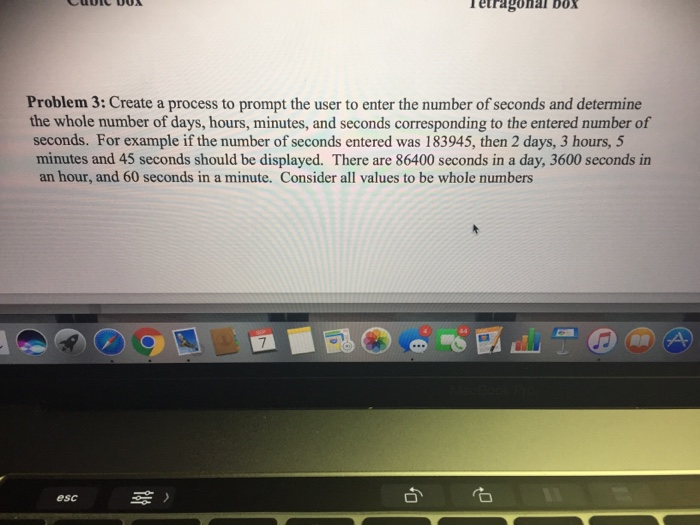How do I program this problem using c++. Create a process to prompt the user to enter the number of seconds and determine the whole number of days, hours, minutes, and seconds corresponding to the entered number of seconds. For example if the number of seconds entered was 183945, then 2 days, 3 hours, 5 minutes and 45 seconds should be displayed. There are 86400 seconds in a day, 3600 seconds in an hour, and 60 seconds in a minute....

• ### Division by Zero Problem: Create a program titled Division. Have the program prompt the user "Enter...

Division by Zero Problem: Create a program titled Division. Have the program prompt the user "Enter numerator and denominator" and store the two values entered. (If you wish the user to "Enter numerator/denominator" be sure to adjust your data gathering variables to include a holder variable for the slash character.) Include a function titled Quotient that takes 2 integers as input and provides the quotient of the 2 integers as integer output. (The remainder is truncated. Thus, 5/3 will be...

• ### Using c++ ) You will write a program that will do the following: prompt the user...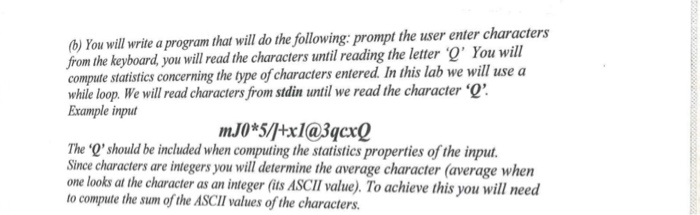Using c++ ) You will write a program that will do the following: prompt the user enter characters from the keyboard you will read the characters until reading the letter O' You will compute statistics concerning the type of characters entered. In this lab we will use a while loop. We will read characters from stdin until we read the character ' Example inpu mJ0*5/tx1@3qcx0 The 'Q'should be included when computing the statistics properties of the input. Since characters are...

• ### In Java please: Write a program that will prompt the user to enter GPA values one...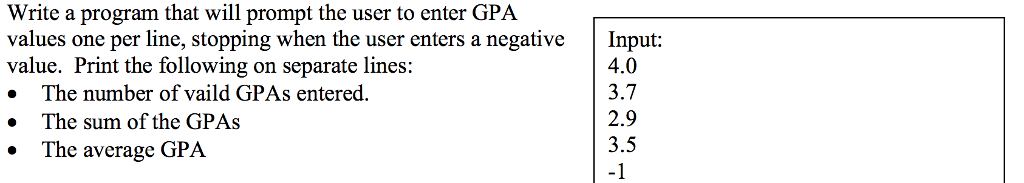In Java please: Write a program that will prompt the user to enter GPA values one per line, stopping when the user enters a negative Input: value. Print the following on separate lines: . The number of vaild GPAs entered » The sum of the GPAs 4.0 3.7 2.9 3.5 -1 The average GPA